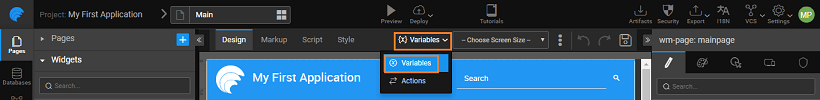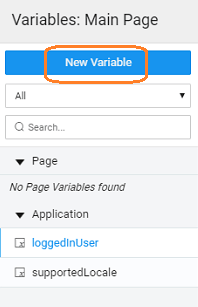Version: v11.5.0

# Model Variable

Model Variable can be used as storage model to store data on the client.

These Variable store data on the page or at the project level. The data can be of various types:

• Boolean value
• Date value
• Entry for name-value pairs
• Number value
• String value
• Database table object
• Service API request or response object

Each of these types can further be in an array format by selecting the isList property.

## Variable Creation​

The data source for these Variables is custom-defined.

1. Select the Variable option from the Variable Workspace Toolbar.1. Click New Variable from the Variable Dialog1. This will initiate Create Variable wizard.

### Steps in Variable Wizard​

1. Select Model as the target action
2. Select the Type based upon the type of data you want the Static variable to hold.
3. Based on the selection, the JSON structure will change
• for default types of  boolean, number, string, date types you will be allowed to enter the values
• for entry type, you can enter the name-dataValue pair
• prebuilt variables gives a pre-populated variable structure - these include country, days, month, and US states list
• for any service types selected, will give you the respective table object structure or request parameters or response format structure
4. Setting the Is List property will allow you to create an array structure of the type selected
5. You can choose to add values using the text editor instead of field editor
6. Data tab will let you set the values dynamically by binding it to a widget or another variable. For example, you can capture the data from a Live Form into an app-level static variable and use it in a different page
7. You will be directed to the Variables page, with the new variable listed. As you can see:
1. a Model Variable is created,
2. the properties tab contains all the properties. Know more about properties.
3. the data tab will contain the fields serving as input fields for the Variable

## Properties​

Static Variables help you store page or application level data. Using Static Variable you can even store data as cookies.

PropertyDescription
TypeProperty to use to specify the data-type of the static variable. This includes live/service variables also.
Is ListProperty to use if the data in the variable is of type array.
JSON
name (for Entry type)Name of the data value in the name-datavalue pair
Data ValueProperty to specify the value to be stored in the variable. You can use either the Field Editor or Text Editor to specify the values.

## Methods​

 getData setData clearData getValue setValue getItem setItem addItem removeItem getCount

### getData()​

This method returns the variable’s dataSet.

Parameters: none

Return Value: If the variable is of list type then returns an Array else it returns an Object

Example:

``var result = Page.Variables.staticVariable1.getData();console.log("result:", result);// Output: // result: {dataValue: “abc”}``

### setData(object)​

This method sets the passed data on the dataSet property of the Variable.

Parameters: Object or Array

Return Value: Newly set dataSet on the variable

Example:

``var result = Page.Variables.staticVariable1.setData({dataValue: “def”});console.log("result:", result);// Output: // result: {dataValue: “def”}``

### getValue(key)​

This method returns the value against the specified key. If dataSet is an array returns the value against the specified key of the object at the specified index.

Parameters:

• key(string):
• index(number): required in case the static variable is an array

Return Value: Value against the key

Example:

``// Example 1: If variable is having dataSet as: {dataValue: “abc”}var result = Page.Variables.staticVariable1.getValue(‘dataValue’);console.log("result:", result);// Output: // result: “abc”// Example 2: If variable is list type having data as:// [{dataValue: “abc”}, {dataValue: “def”}, {dataValue: “ghi”}]var result = Page.Variables.staticVariable1.getValue(‘dataValue’, 1);console.log("result:", result);// Output: // result: “def”``

### setValue(key, value)​

This method sets the value against the specified key.

Parameters:

• key(string)
• value(*)

Return Value: Updated dataSet of variable

Example:

``// Example1: If variable is having dataSet as: {dataValue: “abc”}var result = Page.Variables.staticVariable1.setValue(‘dataValue’, “def”);console.log("result:", result);// Output: // result: {dataValue: “def”}// Example 2: using key parameter. If variable is list type having data as:// [{dataValue: “abc”}, {dataValue: “def”}, {dataValue: “ghi”}]var result = Page.Variables.staticVariable1.setValue('key’, “ghi”);console.log("result:", result);// Output: // result: {dataValue: “def”, key: “ghi”}``

### getItem(index)​

This method returns the object against the specified index.

note

This is only for array type variable, i.e. when the Is List property is set.

Parameters: index(number)

Return Value: Object

Example:

``// If variable is list type having data as:// [{dataValue: “abc”}, {dataValue: “def”}, {dataValue: “ghi”}]var result = Page.Variables.staticVariable1.getItem(1);console.log("result:", result);// Output: // result: {dataValue: “def”}``

### setItem(index, value)​

This method sets the value against the specified index.

note

Only for array type variable, i.e. when the Is List property is set.

Parameters:

• index(number)
• value(*)

Return Value: Updated dataSet of variable

Example:

``// If variable is list type having data as:// [{dataValue: “abc”}, {dataValue: “def”}, {dataValue: “ghi”}]var result = Page.Variables.staticVariable1.setItem(1, {dataValue: "jkl"});console.log("result:", result);// Output: // result: [{dataValue: “abc”}, {dataValue: “jkl”}, {dataValue: “ghi”}]``

This method adds an item at specified index.

note

Only for array type variable, i.e. when the Is List property is set.

Parameters:

• index(number)
• value(*)

Return Value: Updated dataSet of variable

Example:

``//Example1: If variable is list type having data as:// [{dataValue: “abc”}, {dataValue: “def”}, {dataValue: “ghi”}]var result = Page.Variables.staticVariable1.addItem({dataValue: "jkl"});console.log("result:", result);// Output: // result: [{dataValue: “abc”}, {dataValue: “def”}, {dataValue: “ghi”}, {dataValue: “jkl”}]//Example2: If variable is list type having data as:// [{dataValue: “abc”}, {dataValue: “def”}, {dataValue: “ghi”}]var result = Page.Variables.staticVariable1.addItem({dataValue: "jkl"}, 1);console.log("result:", result);// Output: // result: [{dataValue: “abc”}, {dataValue: “jkl”}, {dataValue: “def”}, {dataValue: “ghi”}]``

### removeItem(index)​

This method updates the dataSet of variable

note

Only for array type variable, i.e. when the Is List property is set

Parameters: index(number)

Return Value: Updated dataSet of variable

Example:

``// If variable is list type having data as:// [{dataValue: “abc”}, {dataValue: “def”}, {dataValue: “ghi”}]var result = Page.Variables.staticVariable1.removeItem(1);console.log("result:", result);// Output: // result: [{dataValue: “abc”}, {dataValue: “ghi”}]``

### clearData()​

This method empties the variable dataSet.

note

Only for array type variable, i.e. when the Is List property is set.

Parameters: none

Return Value: Updated dataSet of variable

Example:

``// If variable is list type having data as:// [{dataValue: “abc”}, {dataValue: “def”}, {dataValue: “ghi”}]var result = Page.Variables.staticVariable1.clearData();console.log("result:", result);// Output: // result: []``

### getCount()​

This method returns the total number of items in the dataSet.

note

Only for array type variable, i.e. when the Is List property is set.

Parameters: none

Return Value: Number of items

Example:

``// If variable is list type having data as:// [{dataValue: “abc”}, {dataValue: “def”}, {dataValue: “ghi”}]var result = Page.Variables.staticVariable1.getCount();console.log("result:", result);// Output: // result: 3``